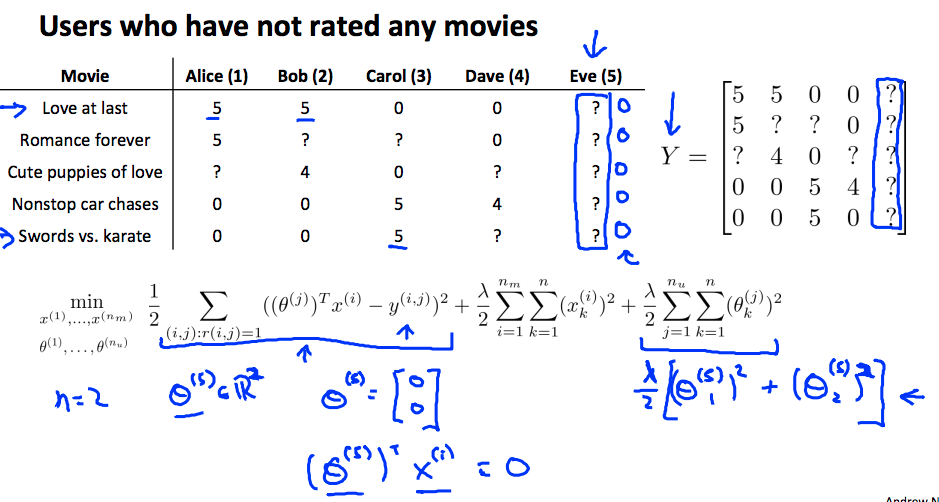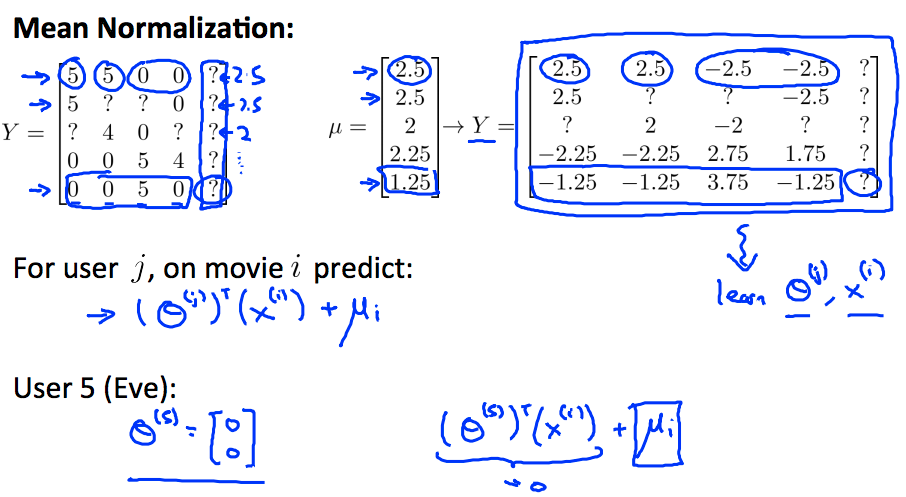# 异常检测

• $x_1 = \thinspace memeory \thinspace use \thinspace of \thinspace the \thinspace computer$
• $x_2 = \thinspace number \thinspace of \thinspace disk \thinspace accesses \thinspace etc$
• $x_3 = \thinspace CPU \thinspace load$
• $x_4 = \thinspace network \thinspace traffic$
• $x_5 = \thinspace CPU \thinspace load \thinspace / \thinspace network \thinspace traffic$
• $x_6 = \thinspace CPU {load^2} \thinspace / \thinspace network \thinspace traffic$

$p\left(x\right)=\frac{1}{\sqrt{2\pi }\sigma }\mathrm{exp}\left(-\frac{\left(x-\mu {\right)}^{2}}{2{\sigma }^{2}}\right)$

• 期望：$\mu =\frac{1}{m}\sum _{i=1}^{m}{x}^{\mathrm{\left(i\right)}}$ 表示样本的均值，极大似然估计值
• 方差：${\sigma }^{2}=\frac{1}{m}\sum _{i=1}^{m}\left({x}^{\mathrm{\left(i\right)}}-\mu {\right)}^{2}$ 表示样本的与均值之间的平均偏差，方差越大，曲线越扁平；方差越小，曲线越陡峭
• 如果某种数据样本符合高斯分布，记作$X~N\left(\mu ,{\sigma }^{2}\right)$，高斯分布也叫做正态分布(Normal Distribution)

$P ( x ) = P ( x1 ; μ1 , σ12 ) P ( x2 ; μ2 , σ22 ) ... P ( xn ; μn , σn2 ) = Π j = 1 n P ( xj ; μj , σj2 )$

• 选取特征数据${x}_{j}$
• 计算每个特征的${\mu }_{1}...{\mu }_{n},{\sigma }_{1}^{2}...{\sigma }_{n}^{2}$ $μj=1m ∑ i = 1 m xj(i)$
$σj2=1m ∑ i = 1 m (xj(i)-μj)2$

Octave 代码如下:

function [mu sigma2] = estimateGaussian(X)

[m, n] = size(X);
mu = zeros(n, 1);
sigma2 = zeros(n, 1);

for i=1:n
mu(i)  = (1/m)*sum(X(:,i));
sigma2(i) =  (1/m)*sum((X(:,i)-mu(i)).^2);
end

end

• 对新的输入样本$x$,计算$p\left(x\right)$，根据阈值判断是否为异常数据$P\left(x\right)<\epsilon$
$P ( x ) = Π j = 1 n P ( xj ; μj , σj2 ) = Π j = 1 n 1 2 π σj exp − ( x − μj ) 2 2 σj2$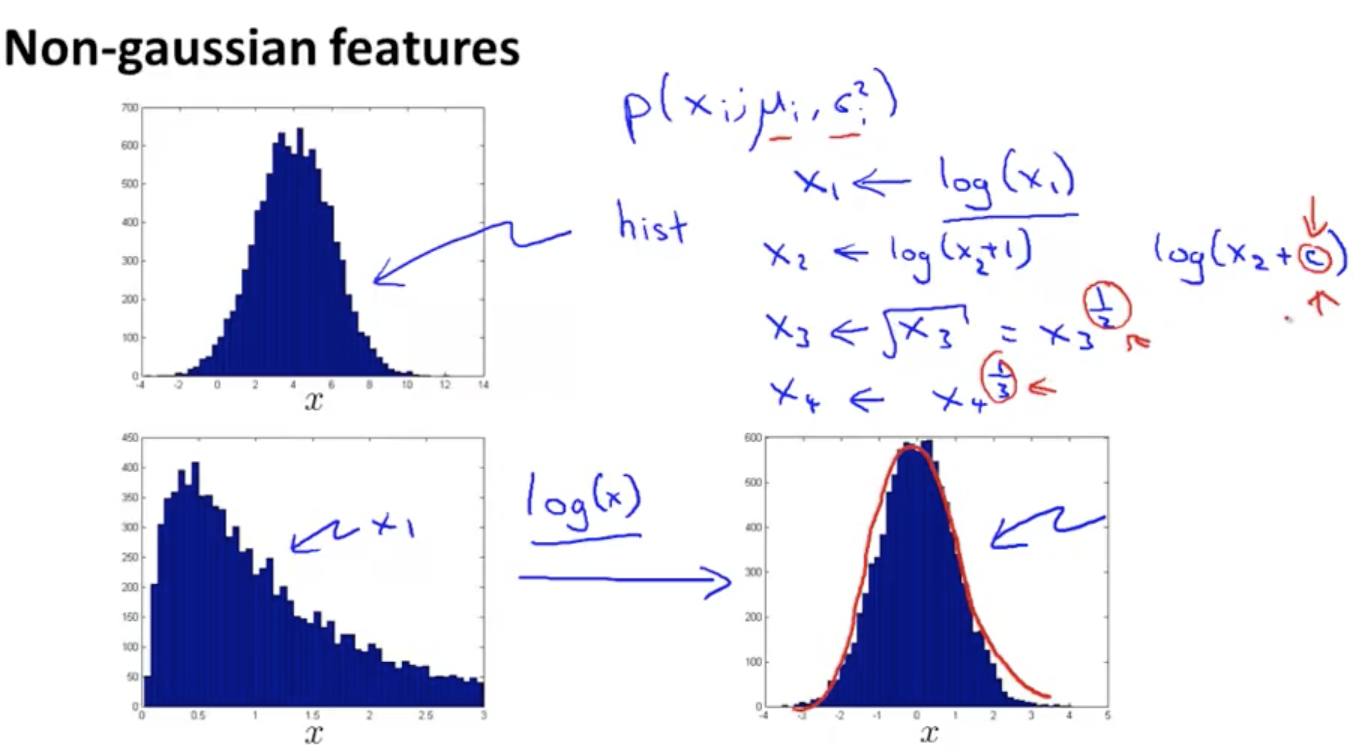• 对于正常的样本数据$x$$p\left(x\right)$值很大
• 对于异常的样本数据$x$$p\left(x\right)$值很小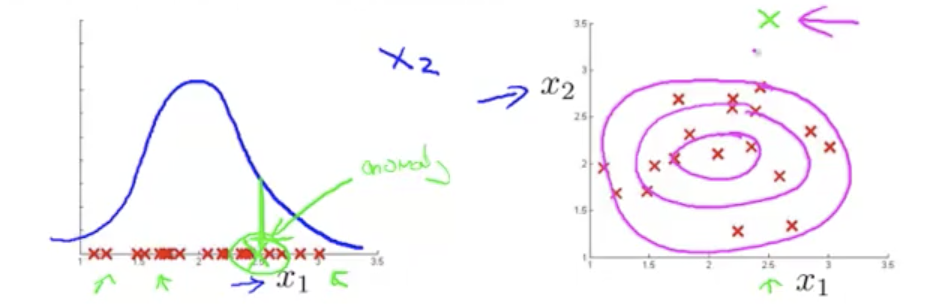$P ( x; μ, ∑ ) = 1 ( 2 π )n/2 |∑|1/2 exp ( - 1 2 ( x - μ ) T ∑ -1 ( x - μ ) )$
• 其中$\mu$为 N 维矩阵，$\mu =\frac{1}{m}\sum _{i=1}^{m}{x}^{\mathrm{\left(i\right)}}$
• $\sum$

为 NxN 协方差矩阵，$\sum =\frac{1}{m}\sum _{i=1}^{m}\left({x}^{\mathrm{\left(i\right)}}-\mu \right)\left({x}^{\mathrm{\left(i\right)}}-\mu {\right)}^{T}$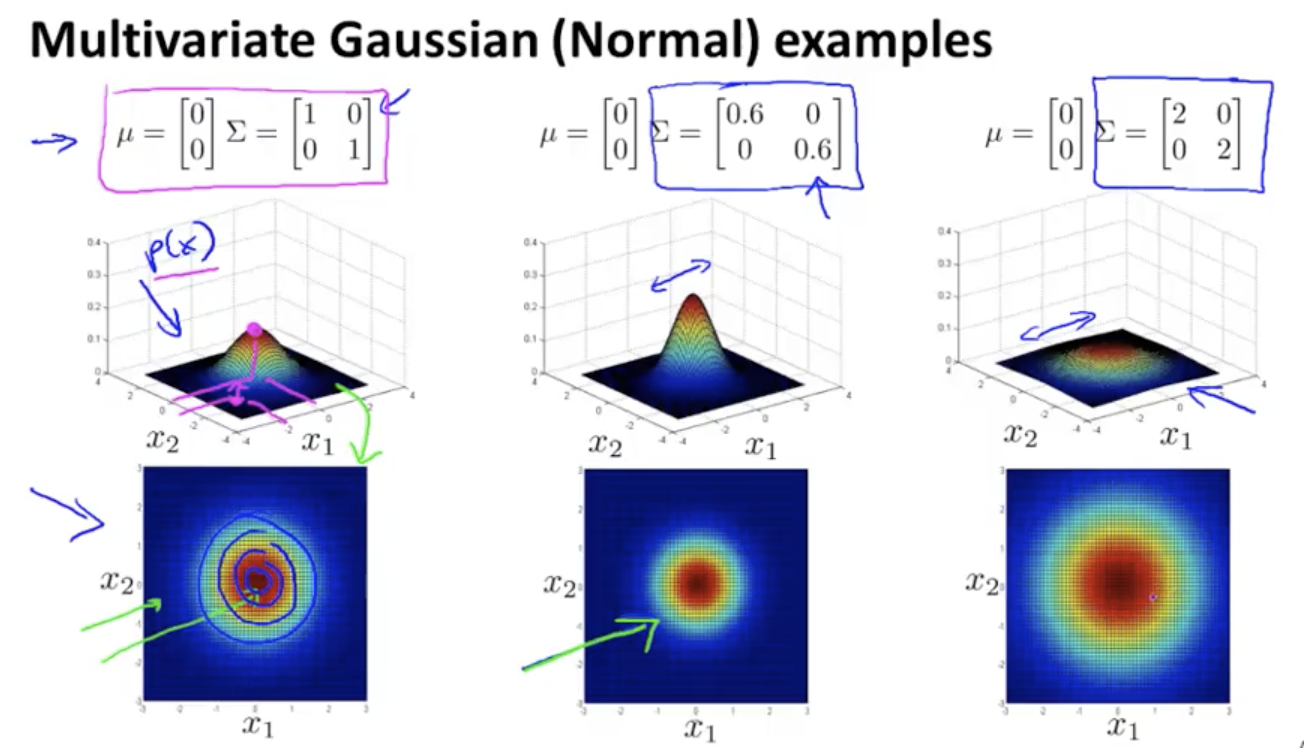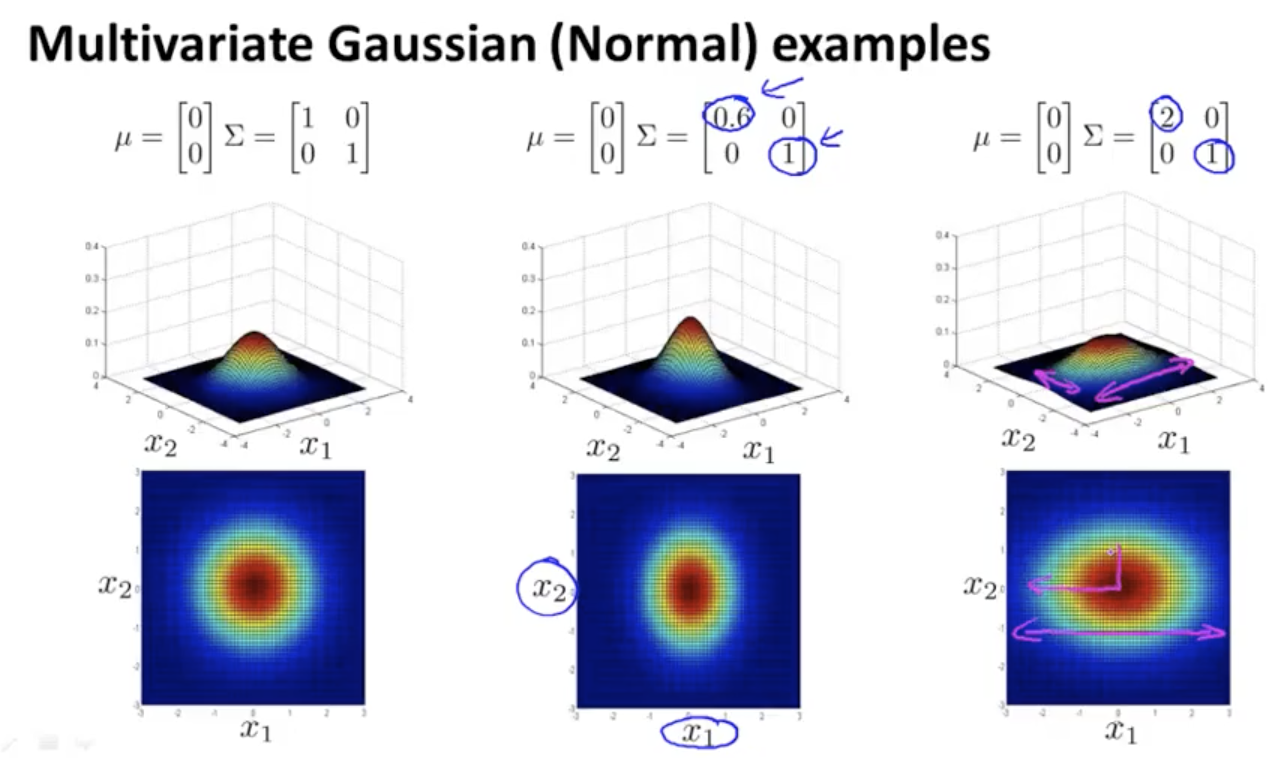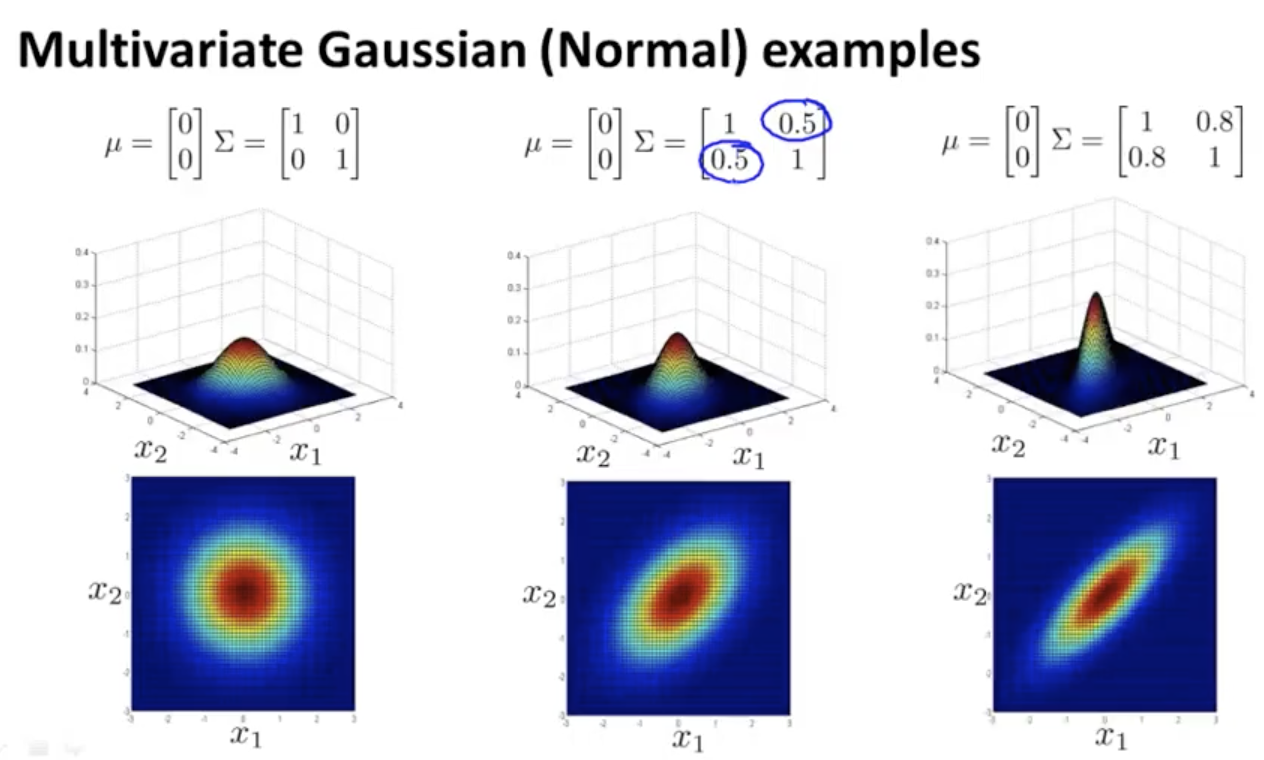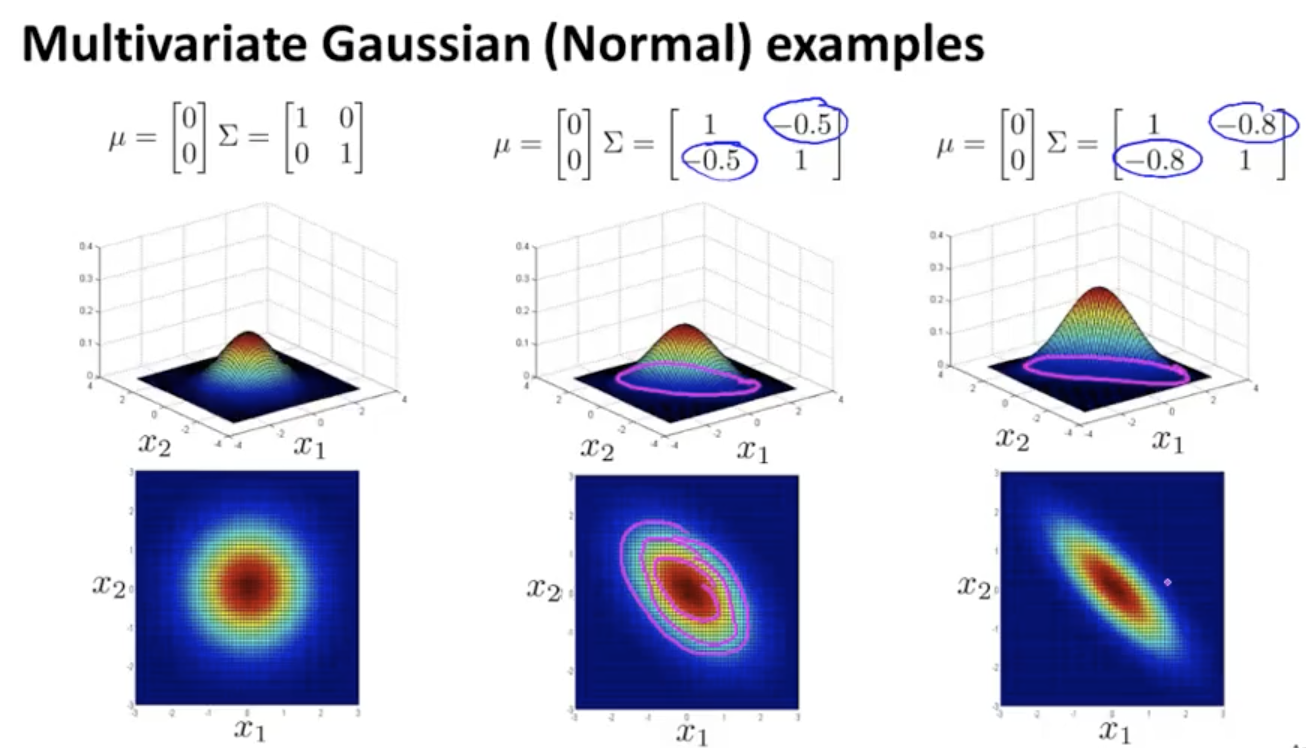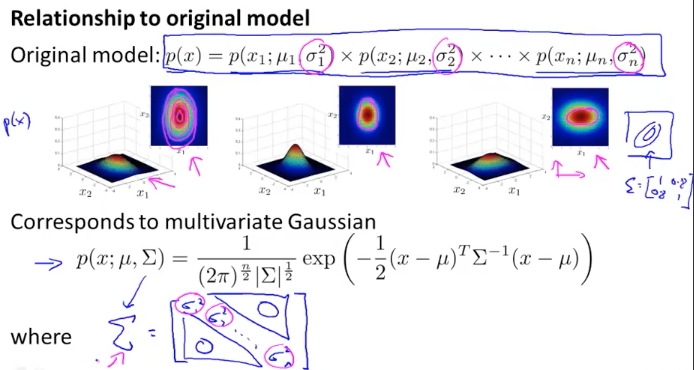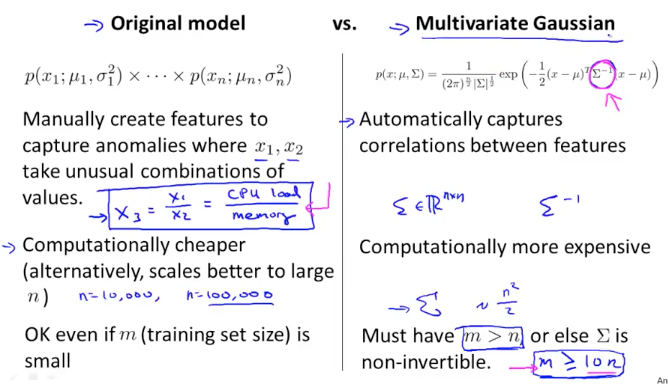### 基于内容的推荐系统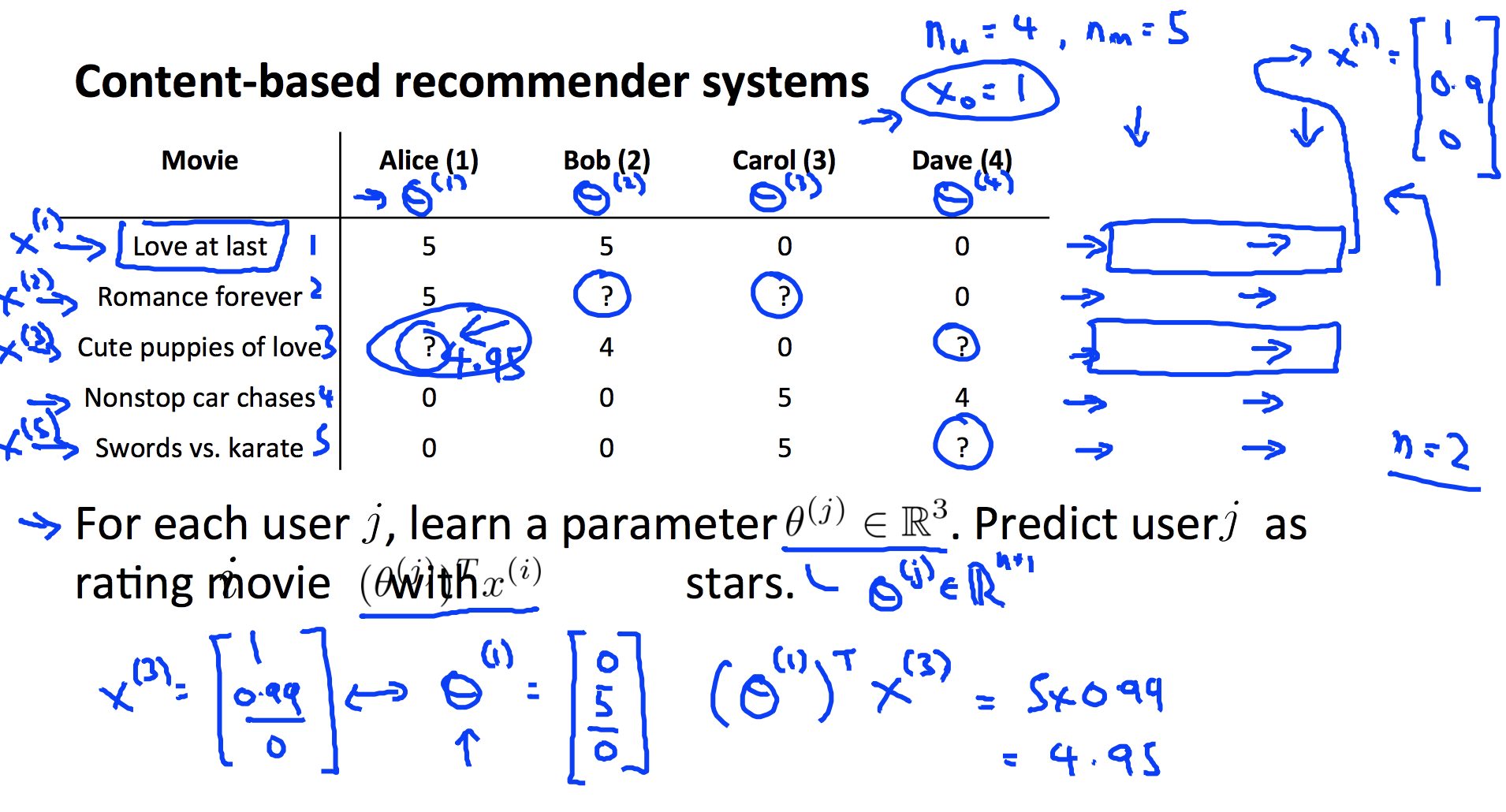• 使用${n}_{u}$表示用户数量，上面例子中${N}_{u}=4$
• 使用${n}_{m}$表示电影数量，上面例子中${N}_{m}=5$
• 使用$r\left(i,j\right)$表示用户$j$是否对电影$i$进行过评分（1 或者 0）
• 使用${y}^{\mathrm{\left(i,j\right)}}$表示用户$j$对电影$i$的评分
• 使用${x}^{\mathrm{\left(i\right)}}$表示电影$i$样本特征向量，其中${x}_{0}=1$，以上面例子第一条数据为例：${x}^{\mathrm{\left(1\right)}}=\left[\begin{array}{c}1\\ 0.9\\ 0\end{array}\right]$
• 使用${\theta }^{\mathrm{\left(j\right)}}$表示用户$j$的参数向量，维度为(N+1)X1
• 则用户对$j$电影$i$的评分的线性模型
$y(i,j) = ( θ(j) ) T x (i)$

$min θ(j) 12 ∑ i:r(i,j)=1 ( ( θ(j) ) T x (i) - y(i,j) )2 + λ2 ∑ k = 1 n ( θk(j) )2$

$min θ(1),...,θnu 12 ∑ j = 1 nu ∑i:r(i,j)=1 ( ( θ(j) ) T x (i) - y(i,j) )2 + λ2 ∑ j = 1 nu ∑ k = 1 n ( θk(j) )2$

$θk(j) := θk(j) - α ∑i:r(i,j)=1 ( ( θ(j) ) T x (i) - y(i,j) ) xk(i) (for k=0)$

$θk(j) := θk(j) - α ( ∑i:r(i,j)=1 ( ( θ(j) ) T x (i) - y(i,j) ) xk(i) + λ θk(j) ) (for k ≠ 0)$

#### 协同过滤(Collaborative Filtering)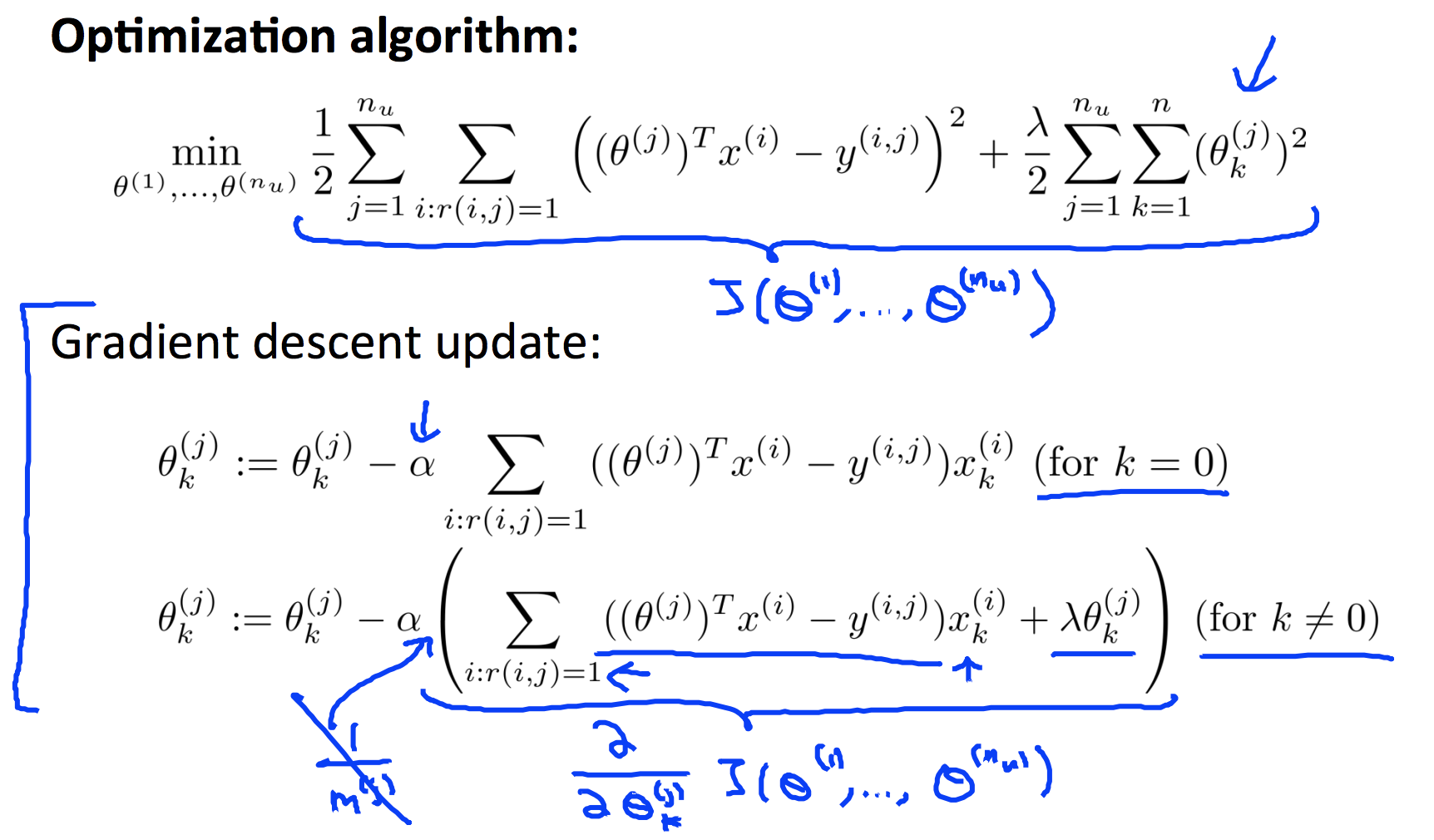$min x(i) 12 ∑j:r(i,j)=1 ( ( θ(j) ) T x (i) - y(i,j) )2 + λ2 ∑ k = 1 n ( xk(i) )2$

$min x(1),...,x(nm) 12 ∑ i = 1 nm ∑i:r(i,j)=1 ( ( θ(j) ) T x (i) - y(i,j) )2 + λ2 ∑ j = 1 nm ∑ k = 1 n ( xk(j) )2$

$xk(i) := xk(i) - α ∑j:r(i,j)=1 ( ( θ(j) ) T x (i) - y(i,j) ) θk(j) (for k=0)$

$xk(i) := xk(i) - α ( ∑j:r(i,j)=1 ( ( θ(j) ) T x (i) - y(i,j) ) θk(j) + λ xk(i) ) (for k ≠ 0)$

$J ( x(1),...,x(nm) , θ(1),...,θ(nu) ) = 12 ∑(i,j):r(i,j)=1 ( ( θ(j) ) T x (i) - y(i,j) )2 + λ2 ∑ j = 1 nm ∑ k = 1 n ( xk(j) )2 + λ2 ∑ j = 1 nu ∑ k = 1 n ( θk(j) )2$

• 初始化${x}^{\mathrm{\left(1\right)}},...,{x}^{\mathrm{\left(nm\right)}}$ , θ(1) , ... , θ(nu)

[/itex]为较小的随机数

• 使用低度下降或其它最优化方法求$J\left({x}^{\mathrm{\left(1\right)}},...,{x}^{\mathrm{\left(nm\right)}},{\theta }^{\mathrm{\left(1\right)}},...,{\theta }^{\mathrm{\left(nu\right)}}\right)$的最小值，得到最优解 θ 和 x,不需要对${\theta }_{0}$${x}_{0}$做特殊处理.
$xk(i) := xk(i) - α ( ∑j:r(i,j)=1 ( ( θ(j) ) T x (i) - y(i,j) ) θk(j) + λ xk(i) )$

$θk(j) := θk(j) - α ( ∑i:r(i,j)=1 ( ( θ(j) ) T x (i) - y(i,j) ) xk(i) + λ θk(j) )$
• 对用户的打分计算使用公式${\theta }^{T}x$

#### 向量化实现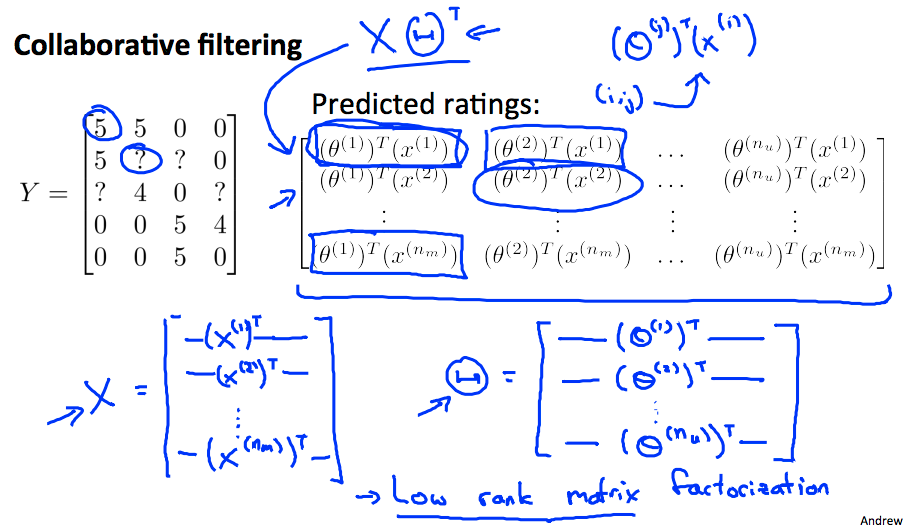$Find smallest || x(i) - x(j) ||$

### 均值归一化